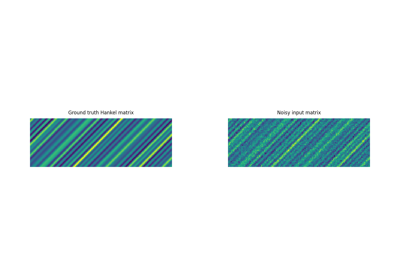Quadratic envelope of the rank function with an L2 misfit term.

The penalty $$p$$ is given by

$p(X) = \mathcal{R}_{r_0}(X) + \frac{1}{2}\|X - M\|_F^2$

where $$\mathcal{R}_{r_0}$$ is the quadratic envelope of the hard-rank function.

Parameters
dimtuple

Size of input matrix $$X$$.

r0int

Threshold parameter, encouraging matrices with rank lower than or equal to r0.

Mnumpy.ndarray

L2 misfit term (must be the same size as the input matrix).

SingularValuePenalty

Proximal operator of a penalty acting on the singular values

QuadraticEnvelopeCardIndicator

Quadratic envelope of the indicator function of $$\ell_0$$-penalty

Notes

The proximal operator solves the minimization problem

$\argmin_Z \mathcal{R}_{r_0}(Z) + \frac{1}{2}\|Z - M\|_F^2 + \frac{1}{2\tau}\| Z - X \|_F^2$

which is a convex-concave min-max problem, see  for details.

References

1

Larsson, V. and Olsson, C. “Convex Low Rank Approximation”, In International Journal of Computer Vision (IJCV), 120:194–214, 2016.

Methods

 __init__(dim, r0, M) affine_addition(v) Affine addition chain(g) Chain grad(x) Compute gradient postcomposition(sigma) Postcomposition precomposition(a, b) Precomposition prox(**kwargs) proxdual(**kwargs)

## Examples using pyproximal.QuadraticEnvelopeRankL2#Hankel matrix estimation

Hankel matrix estimation